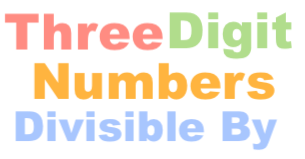Three digit numbers divisible by 14We will start off with a couple of definitions for clarification. Three digit numbers (3-digit numbers) are numbers that have three digits in them. They range from 100 to 999. Therefore, there are a total of 900 3-digit numbers.

Furthermore, a three digit number is divisible by 14 if you divide the three digit number by 14 and you get a whole number with no remainder.

Below you will find many questions and answers related to three digit numbers divisible by 14.

How many three digit numbers are divisible by 14?
Below is a list of all three digit numbers divisible by 14 in chronological order. There are 64 three digit numbers divisible by 14.

112, 126, 140, 154, 168, 182, 196, 210, 224, 238, 252, 266, 280, 294, 308, 322, 336, 350, 364, 378, 392, 406, 420, 434, 448, 462, 476, 490, 504, 518, 532, 546, 560, 574, 588, 602, 616, 630, 644, 658, 672, 686, 700, 714, 728, 742, 756, 770, 784, 798, 812, 826, 840, 854, 868, 882, 896, 910, 924, 938, 952, 966, 980, 994

How many three digit numbers are NOT divisible by 14?
Like we wrote above, there are a total of 900 three digit numbers and 64 of them are divisible by 14. Furthermore, 900 - 64 = 836. Therefore, there are 836 three digit numbers that are not divisible by 14.

What is the sum of all three digit numbers divisible by 14?
We added up all the 3-digit numbers in our list above. The sum of all 3-digit numbers divisible by 14 is 35392.

What is the smallest three digit number divisible by 14?
The smallest or lowest 3-digit number divisible by 14 is the first number on the list above (first 3 digit number divisible by 14). As you can see, that number is 112.

What is the largest three digit number divisible by 14?
The largest or greatest 3-digit number divisible by 14 is the last number on the list above (last 3 digit number divisible by 14). As you can see, that number is 994.

How many even three digit numbers are divisible by 14?
Below is a list of all 3-digit EVEN numbers divisible by 14 in chronological order. There are 64 even three digit numbers divisible by 14.

112, 126, 140, 154, 168, 182, 196, 210, 224, 238, 252, 266, 280, 294, 308, 322, 336, 350, 364, 378, 392, 406, 420, 434, 448, 462, 476, 490, 504, 518, 532, 546, 560, 574, 588, 602, 616, 630, 644, 658, 672, 686, 700, 714, 728, 742, 756, 770, 784, 798, 812, 826, 840, 854, 868, 882, 896, 910, 924, 938, 952, 966, 980, 994

How many odd three digit numbers are divisible by 14?
Below is a list of all 3-digit ODD numbers divisible by 14 in chronological order. There are 0 odd three digit numbers divisible by 14.

None

Three Digit Numbers Divisible By Calculator
There you have it folks! Your are now a "Three digit numbers divisible by 14" expert. You can enter another number below for us to tackle!

Three digit numbers divisible by 15
Here is a similar problem we have explained and answered.